Скачать презентацию Chapter 22 Borrowing Models Lesson Plan n Simple

815faa0b351298e651519df5aae0887d.ppt

• Количество слайдов: 12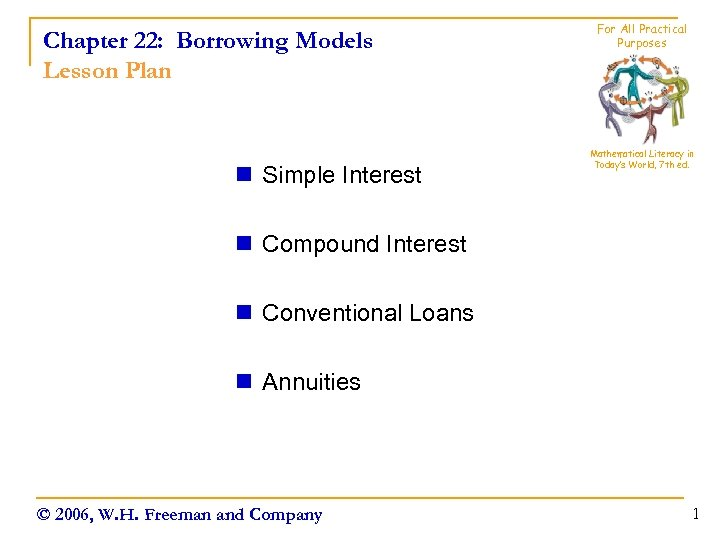Chapter 22: Borrowing Models Lesson Plan n Simple Interest For All Practical Purposes Mathematical Literacy in Today’s World, 7 th ed. n Compound Interest n Conventional Loans n Annuities © 2006, W. H. Freeman and Company 1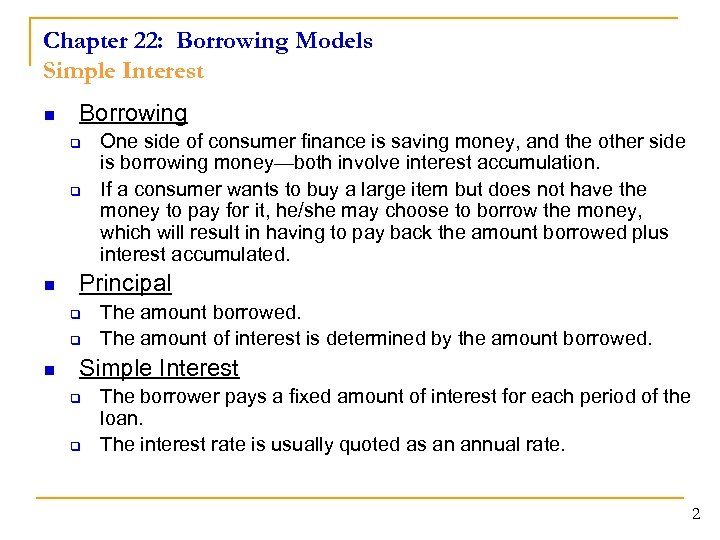Chapter 22: Borrowing Models Simple Interest n Borrowing q q n Principal q q n One side of consumer finance is saving money, and the other side is borrowing money—both involve interest accumulation. If a consumer wants to buy a large item but does not have the money to pay for it, he/she may choose to borrow the money, which will result in having to pay back the amount borrowed plus interest accumulated. The amount borrowed. The amount of interest is determined by the amount borrowed. Simple Interest q q The borrower pays a fixed amount of interest for each period of the loan. The interest rate is usually quoted as an annual rate. 2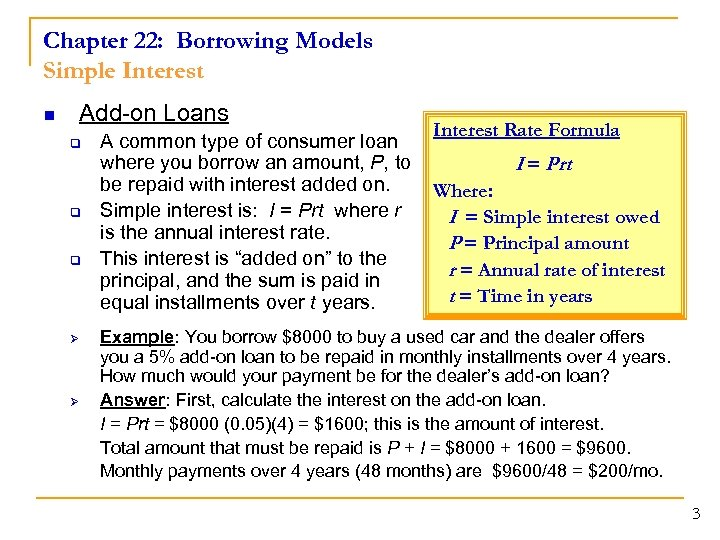Chapter 22: Borrowing Models Simple Interest n Add-on Loans q q q Ø Ø A common type of consumer loan where you borrow an amount, P, to be repaid with interest added on. Simple interest is: I = Prt where r is the annual interest rate. This interest is “added on” to the principal, and the sum is paid in equal installments over t years. Interest Rate Formula I = Prt Where: I = Simple interest owed P = Principal amount r = Annual rate of interest t = Time in years Example: You borrow \$8000 to buy a used car and the dealer offers you a 5% add-on loan to be repaid in monthly installments over 4 years. How much would your payment be for the dealer’s add-on loan? Answer: First, calculate the interest on the add-on loan. I = Prt = \$8000 (0. 05)(4) = \$1600; this is the amount of interest. Total amount that must be repaid is P + I = \$8000 + 1600 = \$9600. Monthly payments over 4 years (48 months) are \$9600/48 = \$200/mo. 3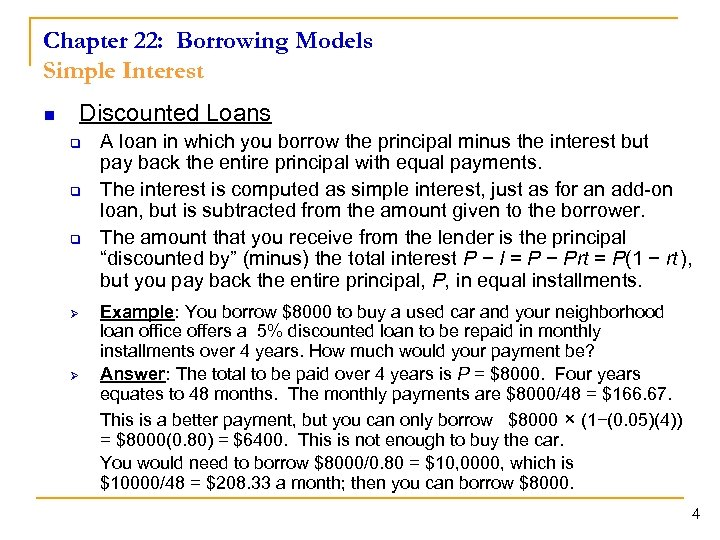Chapter 22: Borrowing Models Simple Interest n Discounted Loans q q q Ø Ø A loan in which you borrow the principal minus the interest but pay back the entire principal with equal payments. The interest is computed as simple interest, just as for an add-on loan, but is subtracted from the amount given to the borrower. The amount that you receive from the lender is the principal “discounted by” (minus) the total interest P − I = P − Prt = P(1 − rt ), but you pay back the entire principal, P, in equal installments. Example: You borrow \$8000 to buy a used car and your neighborhood loan office offers a 5% discounted loan to be repaid in monthly installments over 4 years. How much would your payment be? Answer: The total to be paid over 4 years is P = \$8000. Four years equates to 48 months. The monthly payments are \$8000/48 = \$166. 67. This is a better payment, but you can only borrow \$8000 × (1−(0. 05)(4)) = \$8000(0. 80) = \$6400. This is not enough to buy the car. You would need to borrow \$8000/0. 80 = \$10, 0000, which is \$10000/48 = \$208. 33 a month; then you can borrow \$8000. 4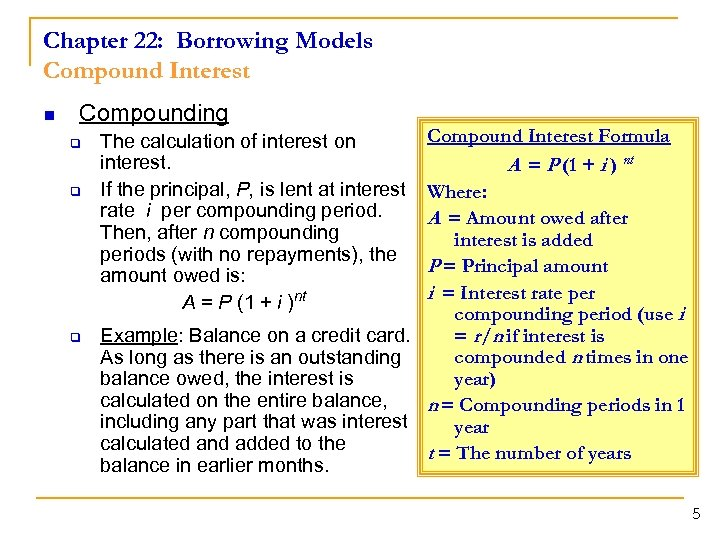Chapter 22: Borrowing Models Compound Interest n Compounding q q q Compound Interest Formula A = P (1 + i ) nt Where: A = Amount owed after interest is added P = Principal amount i = Interest rate per compounding period (use i = r /n if interest is Example: Balance on a credit card. compounded n times in one As long as there is an outstanding year) balance owed, the interest is calculated on the entire balance, n = Compounding periods in 1 including any part that was interest year calculated and added to the t = The number of years balance in earlier months. The calculation of interest on interest. If the principal, P, is lent at interest rate i per compounding period. Then, after n compounding periods (with no repayments), the amount owed is: A = P (1 + i )nt 5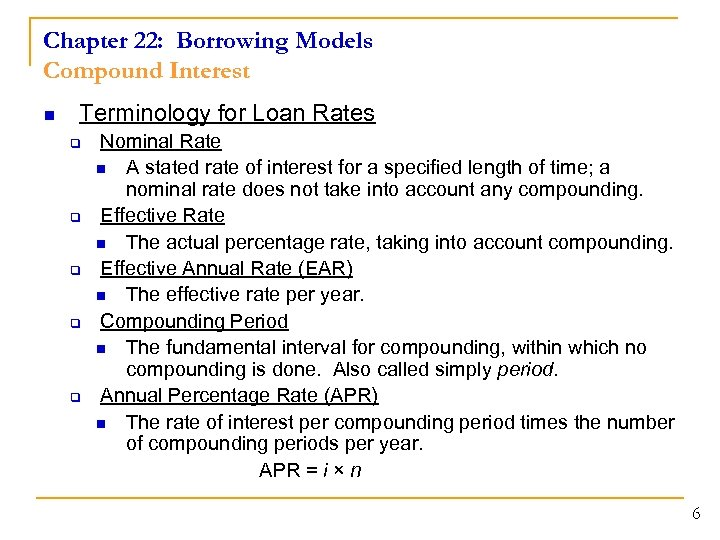Chapter 22: Borrowing Models Compound Interest n Terminology for Loan Rates q q q Nominal Rate n A stated rate of interest for a specified length of time; a nominal rate does not take into account any compounding. Effective Rate n The actual percentage rate, taking into account compounding. Effective Annual Rate (EAR) n The effective rate per year. Compounding Period n The fundamental interval for compounding, within which no compounding is done. Also called simply period. Annual Percentage Rate (APR) n The rate of interest per compounding period times the number of compounding periods per year. APR = i × n 6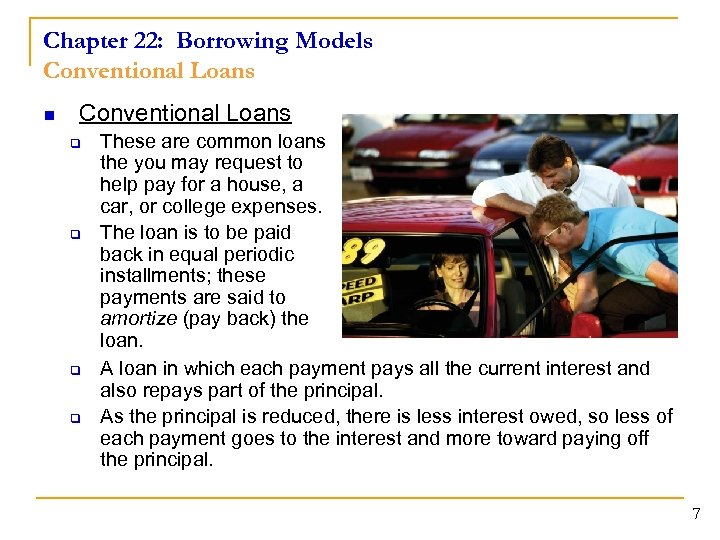Chapter 22: Borrowing Models Conventional Loans n Conventional Loans q q These are common loans the you may request to help pay for a house, a car, or college expenses. The loan is to be paid back in equal periodic installments; these payments are said to amortize (pay back) the loan. A loan in which each payment pays all the current interest and also repays part of the principal. As the principal is reduced, there is less interest owed, so less of each payment goes to the interest and more toward paying off the principal. 7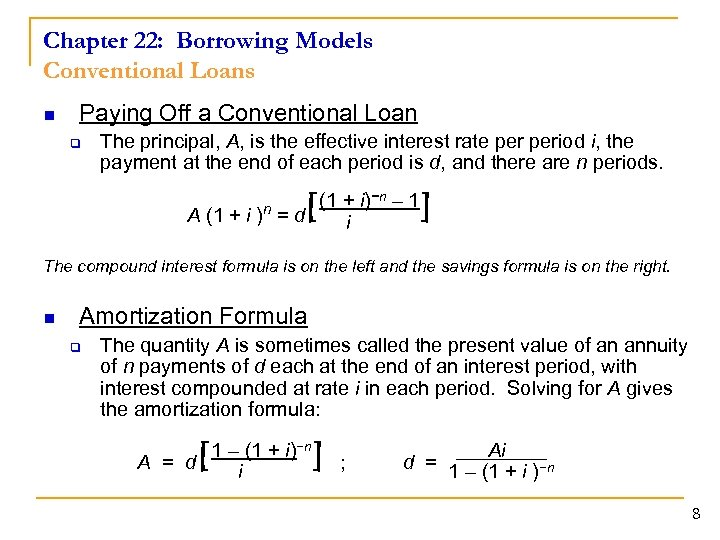Chapter 22: Borrowing Models Conventional Loans n Paying Off a Conventional Loan q The principal, A, is the effective interest rate period i, the payment at the end of each period is d, and there are n periods. (1 + i)−n – 1 A (1 + i ) = d i n [ ] The compound interest formula is on the left and the savings formula is on the right. n Amortization Formula q The quantity A is sometimes called the present value of an annuity of n payments of d each at the end of an interest period, with interest compounded at rate i in each period. Solving for A gives the amortization formula: 1 – (1 + i)−n A = d i [ ] ; Ai d = 1 – (1 + i )−n 8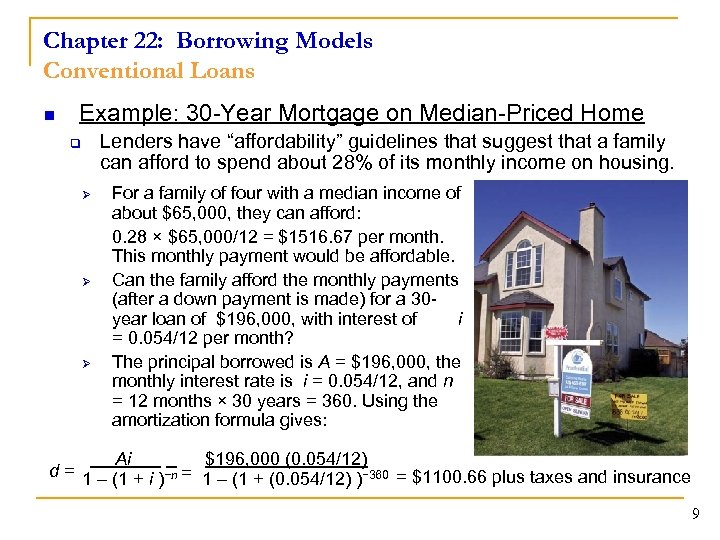Chapter 22: Borrowing Models Conventional Loans n Example: 30 -Year Mortgage on Median-Priced Home Lenders have “affordability” guidelines that suggest that a family can afford to spend about 28% of its monthly income on housing. q Ø Ø Ø For a family of four with a median income of about \$65, 000, they can afford: 0. 28 × \$65, 000/12 = \$1516. 67 per month. This monthly payment would be affordable. Can the family afford the monthly payments (after a down payment is made) for a 30 year loan of \$196, 000, with interest of i = 0. 054/12 per month? The principal borrowed is A = \$196, 000, the monthly interest rate is i = 0. 054/12, and n = 12 months × 30 years = 360. Using the amortization formula gives: Ai. \$196, 000 (0. 054/12) d = 1 – (1 + i )−n = 1 – (1 + (0. 054/12) )− 360 = \$1100. 66 plus taxes and insurance 9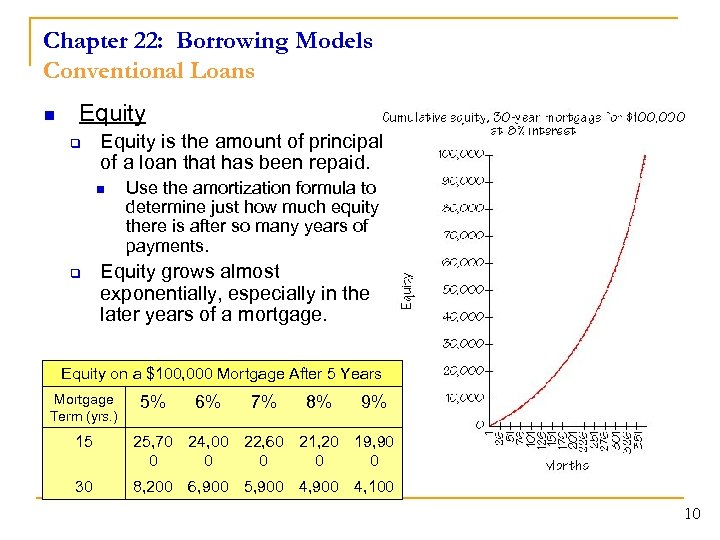Chapter 22: Borrowing Models Conventional Loans n Equity q Equity is the amount of principal of a loan that has been repaid. n q Use the amortization formula to determine just how much equity there is after so many years of payments. Equity grows almost exponentially, especially in the later years of a mortgage. Equity on a \$100, 000 Mortgage After 5 Years Mortgage Term (yrs. ) 5% 6% 7% 8% 9% 15 25, 70 24, 00 22, 60 21, 20 19, 90 0 0 30 8, 200 6, 900 5, 900 4, 100 10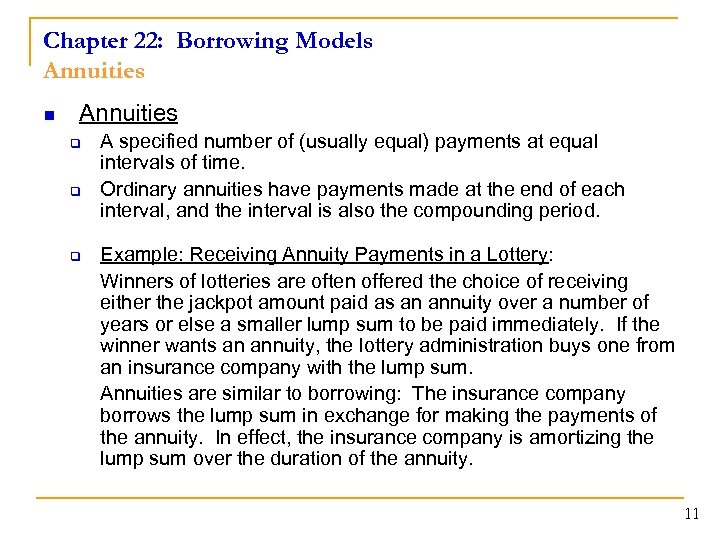Chapter 22: Borrowing Models Annuities n Annuities q q q A specified number of (usually equal) payments at equal intervals of time. Ordinary annuities have payments made at the end of each interval, and the interval is also the compounding period. Example: Receiving Annuity Payments in a Lottery: Winners of lotteries are often offered the choice of receiving either the jackpot amount paid as an annuity over a number of years or else a smaller lump sum to be paid immediately. If the winner wants an annuity, the lottery administration buys one from an insurance company with the lump sum. Annuities are similar to borrowing: The insurance company borrows the lump sum in exchange for making the payments of the annuity. In effect, the insurance company is amortizing the lump sum over the duration of the annuity. 11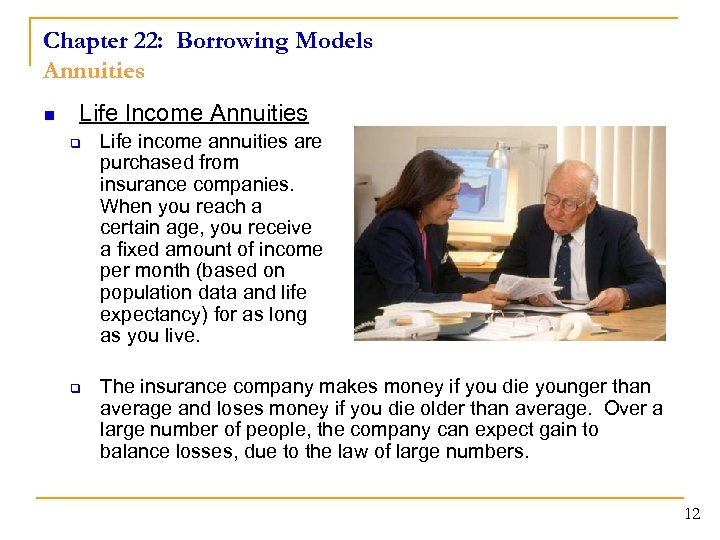Chapter 22: Borrowing Models Annuities n Life Income Annuities q q Life income annuities are purchased from insurance companies. When you reach a certain age, you receive a fixed amount of income per month (based on population data and life expectancy) for as long as you live. The insurance company makes money if you die younger than average and loses money if you die older than average. Over a large number of people, the company can expect gain to balance losses, due to the law of large numbers. 12